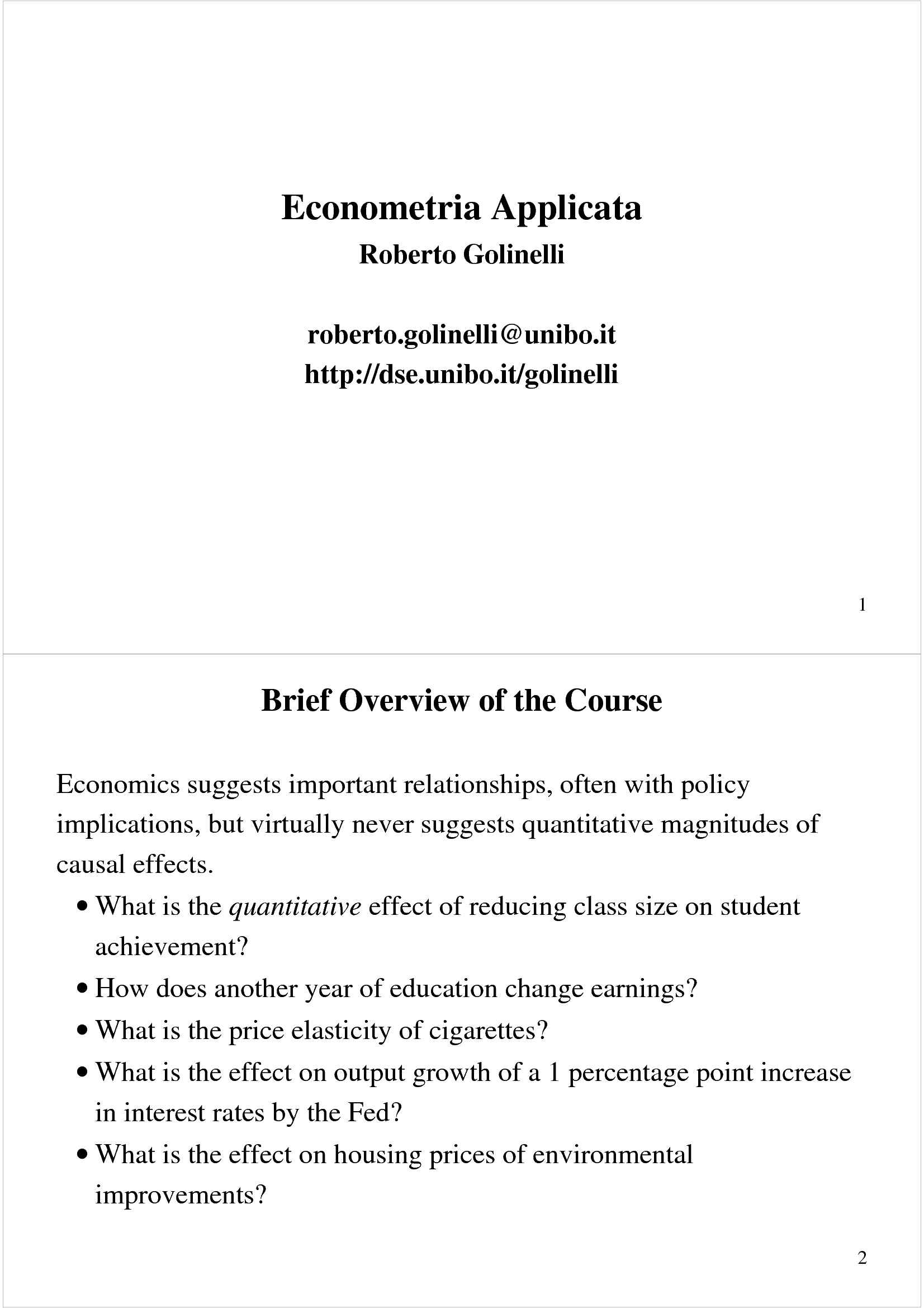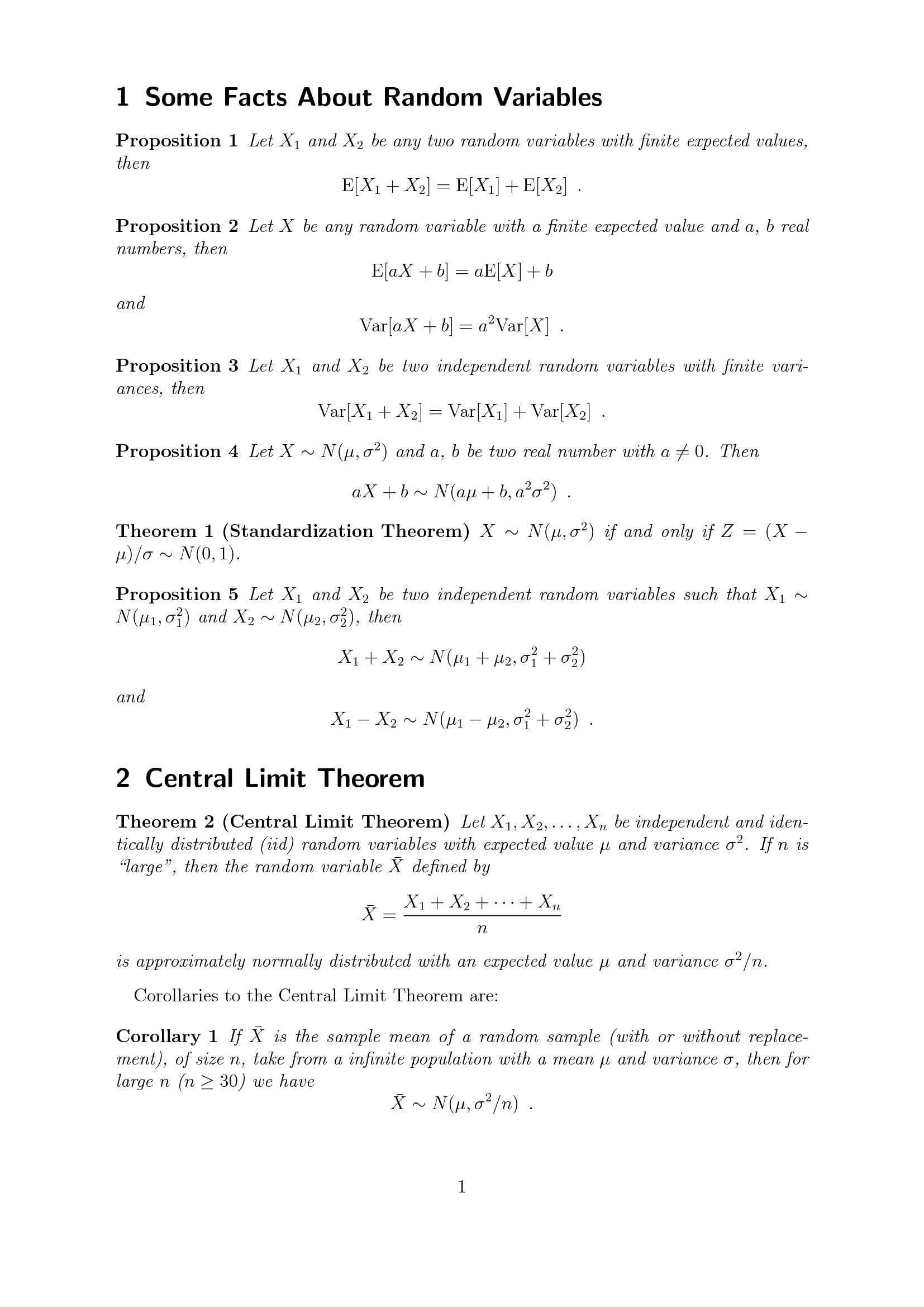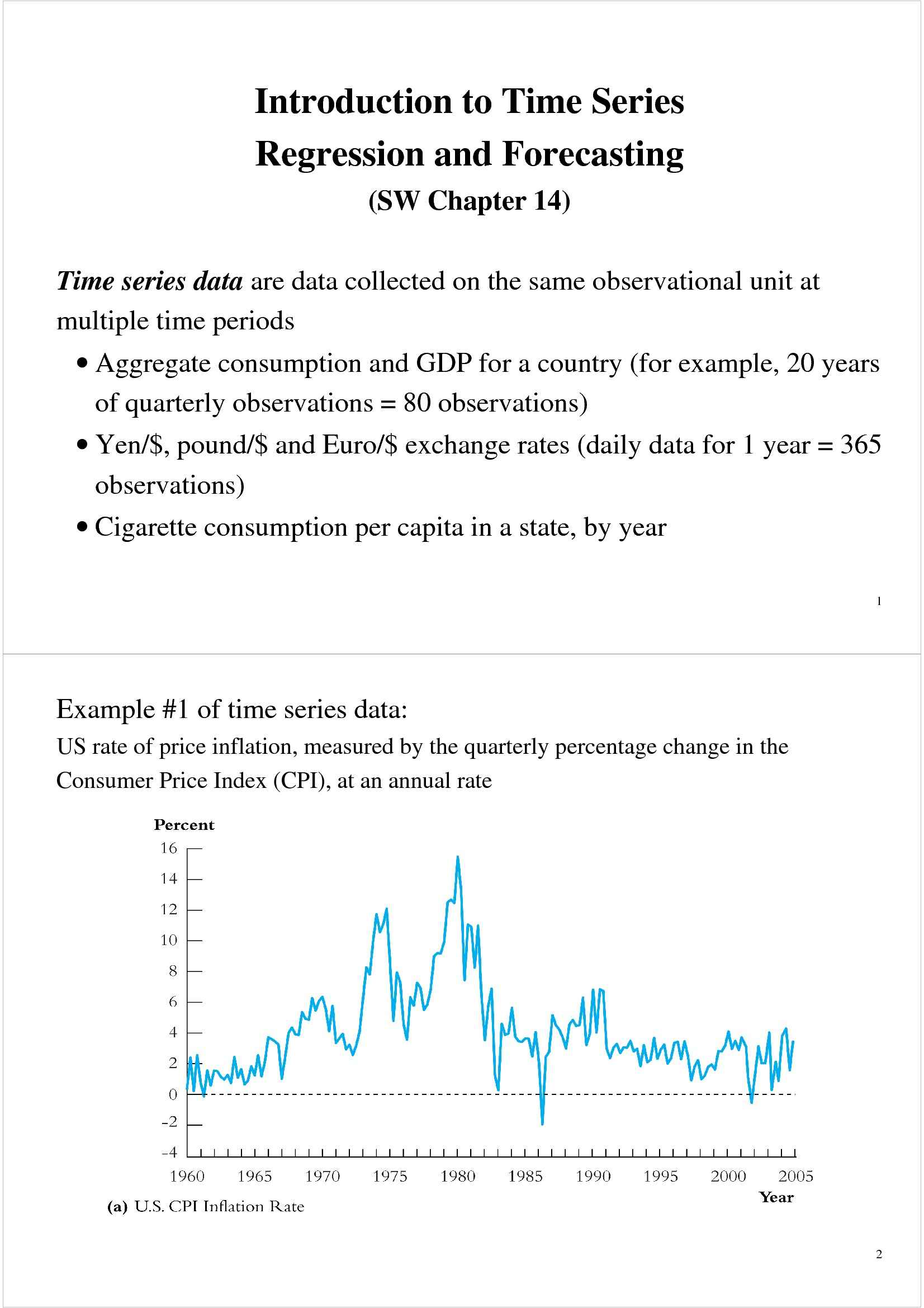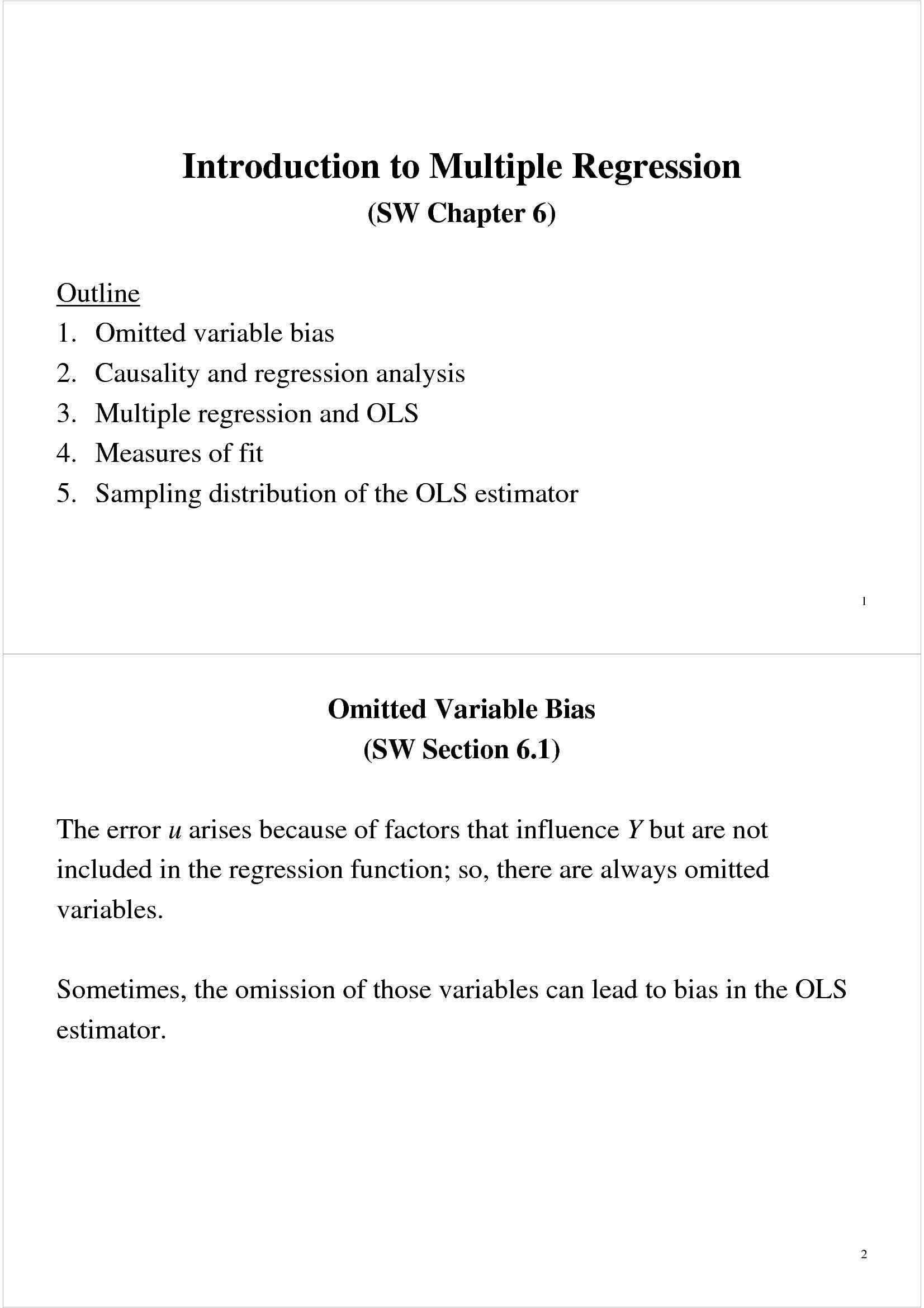Che materia stai cercando?

# Regressione con variabili strumentali

Materiale didattico per il corso di Econometria applicata del prof. Roberto Golinelli. Trattasi di slides in lingua inglese a cura del docente, all'interno delle quali sono affrontati i seguenti argomenti: la regressione con variabili strumentali; variabili esogene e variabili endogene.

Esame di Econometria applicata docente Prof. R. Golinelli

Anteprima

### ESTRATTO DOCUMENTO

This interaction of demand and supply produces…

Would a regression using these data produce the demand curve? 13

But…what would you get if only supply shifted?

• TSLS estimates the demand curve by isolating shifts in price

and quantity that arise from shifts in supply.

• Z is a variable that shifts supply but not demand. 14

TSLS in the supply-demand example:

β β

butter butter

ln( ) = + ln( ) + u

## Q P

0 1 i

i i

Let Z = rainfall in dairy-producing regions.

Is Z a valid instrument? ,u ) = 0?

(1) Exogenous? corr(rain i i

Plausibly: whether it rains in dairy-producing regions

shouldn’t affect demand ≠

butter

(2) Relevant? corr(rain ,ln( P )) 0?

i i

Plausibly: insufficient rainfall means less grazing means

less butter 15

TSLS in the supply-demand example, ctd.

β β

butter butter

) = + ln( ) + u

ln( Q P

0 1 i

i i

Z = rain = rainfall in dairy-producing regions.

i i ˆ butter

ln( P )

butter

P ) on rain, get

Stage 1: regress ln( i

i

ˆ butter

ln( P ) isolates changes in log price that arise from

i

supply (part of supply, at least)

ˆ butter

ln( P )

butter

Q ) on

Stage 2: regress ln( i

i

The regression counterpart of using shifts in the supply

curve to trace out the demand curve. 16

Inference using TSLS

• In large samples, the sampling distribution of the TSLS

estimator is normal

• Inference (hypothesis tests, confidence intervals) proceeds in the

usual way, e.g. ± 1.96×SE

• The idea behind the large-sample normal distribution of the

TSLS estimator is that – like all the other estimators we have

considered – it involves an average of mean zero i.i.d. random

variables, to which we can apply the CLT.

• Here is a sketch of the math (see SW App. 12.3 for the details)...

17

n

1 − −

∑ (

Y Y )( Z Z )

− i i

s n 1

β

ˆ =

TSLS 1

i

## YZ

= =

1 n

1

s − −

XZ ( X X )( Z Z )

− i i

n 1 =

i 1

n −

∑ Y ( Z Z )

i i

=

i 1

= n −

∑ X ( Z Z )

i i

=

i 1

β β

= + X + u and simplify:

Substitute in Y i 0 1 i i

n n

β − + −

∑ ∑

X ( Z Z ) u ( Z Z )

1 i i i i

β = =

ˆ TSLS 1 1

i i

=

1 n −

∑ X ( Z Z )

i i

=

i 1

so… 18

n −

∑ u ( Z Z )

i i

β

β =

ˆ TSLS 1

i

= + .

1

1 n −

∑ X ( Z Z )

i i

=

i 1

n −

∑ u ( Z Z )

i i

β

β =

ˆ TSLS i 1

so – =

1

1 n −

∑ X ( Z Z )

i i

=

i 1

:

Multiply through by n n

1 −

∑ Z Z u

( )

i i

n

β

β =

ˆ TSLS i 1

( – ) =

n 1

1 n

1 −

∑ X Z Z

( )

i i

n =

i 1 19

n

1 −

∑ ( Z Z ) u

i i

n

β

β =

ˆ TSLS i 1

( – ) =

n 1

1 n

1 −

∑ X ( Z Z )

i i

n =

i 1

n n p

1 1

• → ≠

− − −

∑ ∑

= cov(X,Z) 0

( ) ( )( )

## X Z Z X X Z Z

i i i i

n

n = =

i 1 i 1

n

1 µ

• −

∑ is dist’d N(0,var[(Z– )u]) (CLT)

( )

Z Z u Z

i i

n =

i 1 β σ

β

ˆ TSLS 2ˆ

so: is approx. distributed N( , ),

1 β TSLS

1 1

µ

1 var[( ) ]

Z u

σ 2ˆ i Z i

= .

where β TSLS 2

[cov( , )]

n Z X

1 i i

where cov(X,Z) 0 because the instrument is relevant 20

Inference using TSLS, ctd. β σ

β

ˆ 2ˆ

TSLS is approx. distributed N( , ),

1 β TSLS

1 1

• Statistical inference proceeds in the usual way.

• The justification is (as usual) based on large samples

• This all assumes that the instruments are valid – we’ll discuss

what happens if they aren’t valid shortly.

• Important note on standard errors:

The OLS standard errors from the second stage regression

o aren’t right – they don’t take into account the estimation in

ˆ

the first stage ( is estimated).

X i

Instead, use a single specialized command that computes the

o TSLS estimator and the correct SEs.

as usual, use heteroskedasticity-robust SEs

o 21

Example: Cigarette demand, ctd.

β β

cigarettes cigarettes

) = + ln( ) + u

ln( Q P

0 1 i

i i

• Annual cigarette consumption and average prices paid

(including tax)

• n=48 continental US states

Proposed instrumental variable:

• th

= general sales tax per pack in the i state = SalesTax

Z i i

• Is this a valid instrument? ≠

cigarettes

(1) Relevant? corr(SalesTax , ln( P )) 0?

i i

,u ) = 0?

(2) Exogenous? corr(SalesTax

i i 22

Cigarette demand, ctd.

Though panel data, use data from 1995 only.

First stage OLS regression:

ˆ cigarettes

ln( P ) = 4.62 + .031SalesTax , n = 48

i i

Second stage OLS regression:

ˆ ˆ

cigarettes cigarettes

ln(

Q ) ln( P )

= 9.72 – 1.08 , n = 48

i i

Combined regression with correct, heteroskedasticity-robust

standard errors:

ˆ ˆ

cigarettes cigarettes

ln(

Q ) ln( P )

= 9.72 – 1.08 , n = 48

i i

(1.53) (0.32) 23

Summary of IV Regression with a Single X and Z

• A valid instrument Z must satisfy two conditions:

(1) relevance: corr(Z ,X ) 0

i i

(2) exogeneity: corr(Z ,u ) = 0

i i

• , then

TSLS proceeds by first regressing X on Z to get X̂

regressing Y on X̂ .

• The key idea is that the first stage isolates part of the variation in

X that is uncorrelated with u

• If the instrument is valid, then the large-sample sampling

distribution of the TSLS estimator is normal, so inference

proceeds as usual 24

The General IV Regression Model

(SW Section 12.2)

• So far we have considered IV regression with a single

endogenous regressor (X) and a single instrument (Z).

• We need to extend this to:

multiple endogenous regressors (X ,…,X )

o 1 k

multiple included exogenous variables (W ,…,W )

o 1 r

These need to be included for the usual OV reason

,…,Z )

multiple instrumental variables (Z

o 1 m

More (relevant) instruments can produce a smaller variance

2

of TSLS: the R of the first stage increases, so you have

.

more variation in X̂

• Terminology: identification & overidentification 25

Identification

• In general, a parameter is said to be identified if different values

of the parameter would produce different distributions of the

data.

• In IV regression, whether the coefficients are identified depends

on the relation between the number of instruments (m) and the

number of endogenous regressors (k)

• Intuitively, if there are fewer instruments than endogenous

β β

regressors, we can’t estimate ,…,

1 k

For example, suppose k = 1 but m = 0 (no instruments)!

o 26

Identification, ctd.

β β

The coefficients ,…, are said to be:

1 k

• exactly identified if m = k. β β

There are just enough instruments to estimate ,…, .

1 k

• overidentified if m > k. β β

,…, .

There are more than enough instruments to estimate 1 k

If so, you can test whether the instruments are valid (a test of

• underidentified if m < k. β β

There are too few instruments to estimate ,…, . If so, you

1 k

need to get more instruments! 27

The general IV regression model: Summary of jargon

β β β β β

= + X + … + X + W + … + W + u

Y i 0 1 1i k ki k+1 1i k+r ri i

• Y is the dependent variable

i

• X ,…, X are the endogenous regressors (potentially correlated

1i ki

)

with u i

• ,…,W are the included exogenous variables or included

W 1i ri )

exogenous regressors (uncorrelated with u i

β β β

• , ,…, are the unknown regression coefficients

0 1 k+r

• Z ,…,Z are the m instrumental variables (the excluded

1i mi

exogenous variables)

• The coefficients are overidentified if m > k; exactly identified if

m = k; and underidentified if m < k. 28

TSLS with a single endogenous regressor

β β β β

Y = + X + W + … + W + u

i 0 1 1i 2 1i 1+r ri i

• ,…, Z

m instruments: Z 1i m

• First stage

Regress X on all the exogenous regressors: regress X on

o 1 1

W ,…,W , Z ,…, Z by OLS

1 r 1 m ˆ

Compute predicted values , i = 1,…,n

## X

o 1

i

• Second stage

Regress Y on , W ,…, W by OLS

## X̂

o 1 r

1

The coefficients from this second stage regression are the

o TSLS estimators, but SEs are wrong

• To get correct SEs, do this in a single step 29

Example: Demand for cigarettes

β β β

cigarettes cigarettes

) = + ln( ) + ln(Income ) + u

ln( Q P

0 1 2 i i

i i

Z = general sales tax

1i i

= cigarette-specific tax

Z 2i i

• cigarettes

Endogenous variable: ln( P ) (“one X”)

i

• ) (“one W”)

Included exogenous variable: ln(Income

i

• Instruments (excluded endogenous variables): general sales tax,

cigarette-specific tax (“two Zs”)

β

• Is the demand elasticity overidentified, exactly identified, or

1

underidentified? 30

TSLS estimates, Z = sales tax (m = 1)

ˆ ˆ

cigarettes cigarettes

ln(

Q ) ln( P )

= 9.43 – 1.14 + 0.21 ln(Income )

i i i

(0.31)

(1.26) (0.37)

TSLS estimates, Z = sales tax, cig-only tax (m = 2)

ˆ ˆ

cigarettes cigarettes

ln(

Q ) ln( P )

= 9.89 – 1.28 + 0.28 ln(Income )

i i i

(0.96) (0.25) (0.25)

• Smaller SEs for m = 2. Using 2 instruments gives more

information – more “as-if random variation”.

• Low income elasticity (not a luxury good); income elasticity not

statistically significantly different from 0

• Surprisingly high price elasticity 31

The General Instrument Validity Assumptions

β β β β β

= + X + … + X + W + … + W + u

Y i 0 1 1i k ki k+1 1i k+r ri i

(1) Instrument exogeneity: corr(Z ,u ) = 0,…, corr(Z ,u ) = 0

1i i mi i

(2) Instrument relevance: General case, multiple X’s

Suppose the second stage regression could be run using the

predicted values from the population first stage regression.

Then: there is no perfect multicollinearity in this (infeasible)

second stage regression.

• Multicollinearity interpretation…

• Special case of one X: the general assumption is equivalent

to (a) at least one instrument must enter the population

counterpart of the first stage regression, and (b) the W’s are

not perfectly multicollinear. 32

The IV Regression Assumptions

β β β β β

Y = + X + … + X + W + … + W + u

i 0 1 1i k ki k+1 1i k+r ri i

1. E(u |W ,…,W ) = 0

i 1i ri

• #1 says “the exogenous regressors are exogenous.”

2. (Y ,X ,…,X ,W ,…,W ,Z ,…,Z ) are i.i.d.

i 1i ki 1i ri 1i mi

• #2 is not new th moments

3. The X’s, W’s, Z’s, and Y have nonzero, finite 4

• #3 is not new

4. The instruments (Z ,…,Z ) are valid.

1i mi

• We have discussed this

• Under 1-4, TSLS and its t-statistic are normally distributed

• The critical requirement is that the instruments be valid… 33

Checking Instrument Validity

(SW Section 12.3)

Recall the two requirements for valid instruments:

1. Relevance (special case of one X)

At least one instrument must enter the population counterpart

of the first stage regression.

2. Exogeneity

All the instruments must be uncorrelated with the error term:

,u ) = 0,…, corr(Z ,u ) = 0

corr(Z 1i i mi i

What happens if one of these requirements isn’t satisfied? How

can you check? What do you do?

If you have multiple instruments, which should you use? 34

PAGINE

26

PESO

451.44 KB

AUTORE

PUBBLICATO

+1 anno fa

DETTAGLI
Corso di laurea: Corso di laurea in economia, mercati e istituzioni
SSD:
Università: Bologna - Unibo
A.A.: 2011-2012

I contenuti di questa pagina costituiscono rielaborazioni personali del Publisher Atreyu di informazioni apprese con la frequenza delle lezioni di Econometria applicata e studio autonomo di eventuali libri di riferimento in preparazione dell'esame finale o della tesi. Non devono intendersi come materiale ufficiale dell'università Bologna - Unibo o del prof Golinelli Roberto.

Acquista con carta o conto PayPal

Scarica il file tutte le volte che vuoi

Paga con un conto PayPal per usufruire della garanzia Soddisfatto o rimborsato

RecensioniTi è piaciuto questo appunto? Valutalo!

## Altri appunti di Econometria applicataDispensa

### Econometria - elementiDispensa

### Riepilogo di concetti statisticiDispensa

### Regressione forecasting e time seriesDispensa